# 4.8 Fitting exponential models to data  (Page 8/12)

 Page 8 / 12

Use the regression feature to find an exponential function that best fits the data in the table.

$f\left(x\right)=731.92{\left(0.738\right)}^{x}$

Write the exponential function as an exponential equation with base $\text{\hspace{0.17em}}e.$

Graph the exponential equation on the scatter diagram.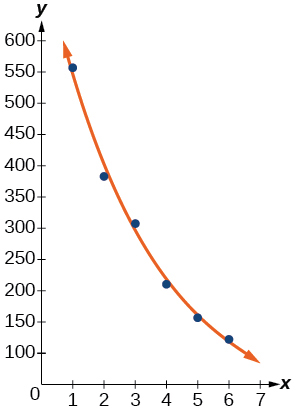Use the intersect feature to find the value of $\text{\hspace{0.17em}}x\text{\hspace{0.17em}}$ for which $\text{\hspace{0.17em}}f\left(x\right)=250.$

For the following exercises, refer to [link] .

 x f(x) 1 5.1 2 6.3 3 7.3 4 7.7 5 8.1 6 8.6

Use a graphing calculator to create a scatter diagram of the data.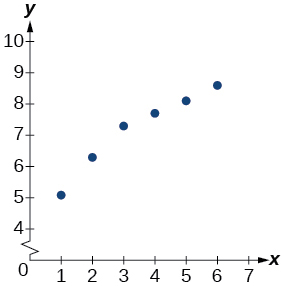Use the LOGarithm option of the REGression feature to find a logarithmic function of the form $\text{\hspace{0.17em}}y=a+b\mathrm{ln}\left(x\right)\text{\hspace{0.17em}}$ that best fits the data in the table.

Use the logarithmic function to find the value of the function when $\text{\hspace{0.17em}}x=10.$

$f\left(10\right)\approx 9.5$

Graph the logarithmic equation on the scatter diagram.

Use the intersect feature to find the value of $\text{\hspace{0.17em}}x\text{\hspace{0.17em}}$ for which $\text{\hspace{0.17em}}f\left(x\right)=7.$

When $\text{\hspace{0.17em}}f\left(x\right)=7,$ $x\approx 2.7.$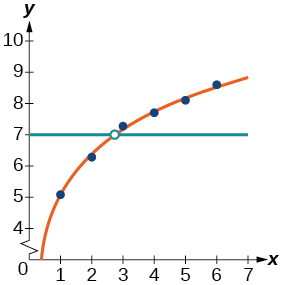For the following exercises, refer to [link] .

 x f(x) 1 7.5 2 6 3 5.2 4 4.3 5 3.9 6 3.4 7 3.1 8 2.9

Use a graphing calculator to create a scatter diagram of the data.

Use the LOGarithm option of the REGression feature to find a logarithmic function of the form $\text{\hspace{0.17em}}y=a+b\mathrm{ln}\left(x\right)\text{\hspace{0.17em}}$ that best fits the data in the table.

$f\left(x\right)=7.544-2.268\mathrm{ln}\left(x\right)$

Use the logarithmic function to find the value of the function when $\text{\hspace{0.17em}}x=10.$

Graph the logarithmic equation on the scatter diagram.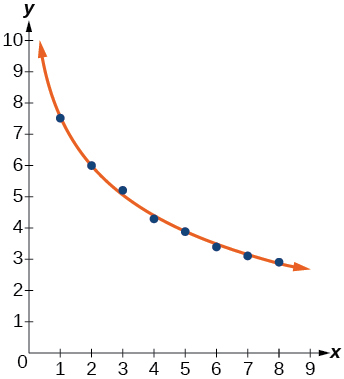Use the intersect feature to find the value of $\text{\hspace{0.17em}}x\text{\hspace{0.17em}}$ for which $\text{\hspace{0.17em}}f\left(x\right)=8.$

For the following exercises, refer to [link] .

 x f(x) 1 8.7 2 12.3 3 15.4 4 18.5 5 20.7 6 22.5 7 23.3 8 24 9 24.6 10 24.8

Use a graphing calculator to create a scatter diagram of the data.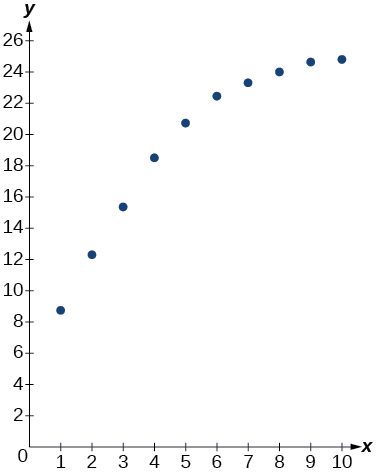Use the LOGISTIC regression option to find a logistic growth model of the form $\text{\hspace{0.17em}}y=\frac{c}{1+a{e}^{-bx}}\text{\hspace{0.17em}}$ that best fits the data in the table.

Graph the logistic equation on the scatter diagram.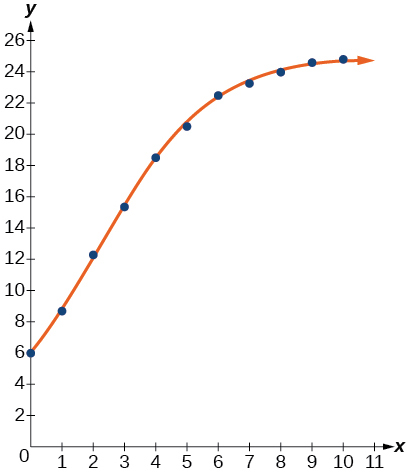To the nearest whole number, what is the predicted carrying capacity of the model?

Use the intersect feature to find the value of $\text{\hspace{0.17em}}x\text{\hspace{0.17em}}$ for which the model reaches half its carrying capacity.

When $\text{\hspace{0.17em}}f\left(x\right)=12.5,$ $x\approx 2.1.$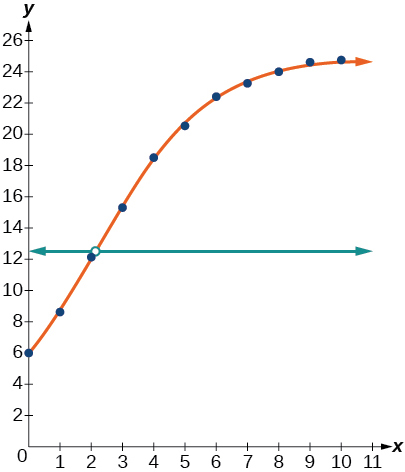For the following exercises, refer to [link] .

 $x$ $f\left(x\right)$ 0 12 2 28.6 4 52.8 5 70.3 7 99.9 8 112.5 10 125.8 11 127.9 15 135.1 17 135.9

Use a graphing calculator to create a scatter diagram of the data.

Use the LOGISTIC regression option to find a logistic growth model of the form $\text{\hspace{0.17em}}y=\frac{c}{1+a{e}^{-bx}}\text{\hspace{0.17em}}$ that best fits the data in the table.

$f\left(x\right)=\frac{136.068}{1+10.324{e}^{-0.480x}}$

Graph the logistic equation on the scatter diagram.

To the nearest whole number, what is the predicted carrying capacity of the model?

about $136$

Use the intersect feature to find the value of $\text{\hspace{0.17em}}x\text{\hspace{0.17em}}$ for which the model reaches half its carrying capacity.

## Extensions

Recall that the general form of a logistic equation for a population is given by $\text{\hspace{0.17em}}P\left(t\right)=\frac{c}{1+a{e}^{-bt}},$ such that the initial population at time $\text{\hspace{0.17em}}t=0\text{\hspace{0.17em}}$ is $\text{\hspace{0.17em}}P\left(0\right)={P}_{0}.\text{\hspace{0.17em}}$ Show algebraically that $\text{\hspace{0.17em}}\frac{c-P\left(t\right)}{P\left(t\right)}=\frac{c-{P}_{0}}{{P}_{0}}{e}^{-bt}.$

Working with the left side of the equation, we see that it can be rewritten as $\text{\hspace{0.17em}}a{e}^{-bt}:$

$\frac{c-P\left(t\right)}{P\left(t\right)}=\frac{c-\frac{c}{1+a{e}^{-bt}}}{\frac{c}{1+a{e}^{-bt}}}=\frac{\frac{c\left(1+a{e}^{-bt}\right)-c}{1+a{e}^{-bt}}}{\frac{c}{1+a{e}^{-bt}}}=\frac{\frac{c\left(1+a{e}^{-bt}-1\right)}{1+a{e}^{-bt}}}{\frac{c}{1+a{e}^{-bt}}}=1+a{e}^{-bt}-1=a{e}^{-bt}$

Working with the right side of the equation we show that it can also be rewritten as $\text{\hspace{0.17em}}a{e}^{-bt}.\text{\hspace{0.17em}}$ But first note that when $\text{\hspace{0.17em}}t=0,$ $\text{\hspace{0.17em}}{P}_{0}=\frac{c}{1+a{e}^{-b\left(0\right)}}=\frac{c}{1+a}.\text{\hspace{0.17em}}$ Therefore,

$\frac{c-{P}_{0}}{{P}_{0}}{e}^{-bt}=\frac{c-\frac{c}{1+a}}{\frac{c}{1+a}}{e}^{-bt}=\frac{\frac{c\left(1+a\right)-c}{1+a}}{\frac{c}{1+a}}{e}^{-bt}=\frac{\frac{c\left(1+a-1\right)}{1+a}}{\frac{c}{1+a}}{e}^{-bt}=\left(1+a-1\right){e}^{-bt}=a{e}^{-bt}$

Thus, $\text{\hspace{0.17em}}\frac{c-P\left(t\right)}{P\left(t\right)}=\frac{c-{P}_{0}}{{P}_{0}}{e}^{-bt}.$

how fast can i understand functions without much difficulty
what is set?
a colony of bacteria is growing exponentially doubling in size every 100 minutes. how much minutes will it take for the colony of bacteria to triple in size
I got 300 minutes. is it right?
Patience
no. should be about 150 minutes.
Jason
It should be 158.5 minutes.
Mr
ok, thanks
Patience
100•3=300 300=50•2^x 6=2^x x=log_2(6) =2.5849625 so, 300=50•2^2.5849625 and, so, the # of bacteria will double every (100•2.5849625) = 258.49625 minutes
Thomas
what is the importance knowing the graph of circular functions?
can get some help basic precalculus
What do you need help with?
Andrew
how to convert general to standard form with not perfect trinomial
can get some help inverse function
ismail
Rectangle coordinate
how to find for x
it depends on the equation
Robert
yeah, it does. why do we attempt to gain all of them one side or the other?
Melissa
whats a domain
The domain of a function is the set of all input on which the function is defined. For example all real numbers are the Domain of any Polynomial function.
Spiro
Spiro; thanks for putting it out there like that, 😁
Melissa
foci (–7,–17) and (–7,17), the absolute value of the differenceof the distances of any point from the foci is 24.
difference between calculus and pre calculus?
give me an example of a problem so that I can practice answering
x³+y³+z³=42
Robert
dont forget the cube in each variable ;)
Robert
of she solves that, well ... then she has a lot of computational force under her command ....
Walter
what is a function?
I want to learn about the law of exponent
explain this

#### Get Jobilize Job Search Mobile App in your pocket Now!ByByBy OpenStaxBy Brooke DelaneyBy OpenStaxBy Yasser IbrahimBy Anh DaoBy OpenStaxBy Sean WiffleBoyBy OpenStaxBy OpenStaxBy Cath Yu